基于Pareto遗传算法和TRIZ理论的数控加工参数优化Optimization of CNC Machining Parameter Based on Pareto Genetic Algorithm and TRIZ Theory

• 全文下载: PDF(1385KB)    PP.419-428   DOI: 10.12677/MET.2018.76051
• 下载量: 272  浏览量: 479

Based on the CNC machine parameter optimization, an algorithm was proposed of Pareto genetic algorithm and TRIZ theory. First, a multi-objective optimization model was built of cutting efficiency, tool life and processing cost as the optimization objectives, and the Pareto optimal solutions were generated based on the Pareto genetic algorithm. Second, based on TRIZ theory, technical contradiction was analyzed on the Pareto optimal solutions and contradiction matrix was built, and the optimal solution was decided based on technical problem-solving principles; at last, this algorithm was proved feasible and effective by experiments results. This method effectively avoids the drawbacks of experience and preference, and the good combination of reasonable optimization and rational decision was achieved.

1. 引言

2. 改进型Pareto遗传算法

TRIZ能够有效解决产品创新中遇到的问题，解决矛盾和冲突是产品创新的核心  ，科学运用RIZ理论中的矛盾解决策略能够有效消除产品创新过程中出现的技术冲突和物理冲突。TRIZ理论提出了39个工程参数的抽象描述方法，而且这些描述方法能够有效解释对象冲突的本质，TRIZ理论还提出了39个通用的工程参数，构建了用于分析参数问题的冲突矩阵模型，其中“列”所描述的是需要改善的一方，而“行”所针对的则是恶化的一方，而后基于一定的规则，将40条发明原理视为参考解列入其中，从而得到冲突解决原理表。在实际应用过程中，为了促使问题实现抽象化，还需要借助参数来进行定义描述，并在矩阵表中确定对应的原理号  ，根据解决原理号及原理内容，结合实际，分析、提炼和总结，最终找到解决问题的方法。TRIZ理论的39个通用工程参数及其40条发明原理是针对所有问题基础上的提炼和通用，从而保证了应用的科学性。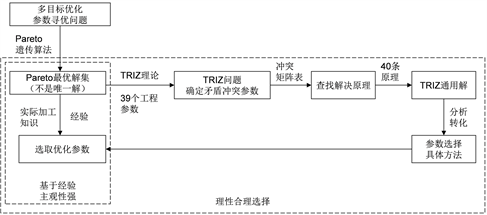Figure 1. Improved Pareto genetic algorithm flow chart

3. 建立数学模型

3.1. 设计变量

3.2. 目标函数

$E\left(v,f\right)=\frac{1}{{t}_{m}}=\frac{1000vfZ}{\pi dL}$ (1)

$T\left(v,f\right)=\frac{{C}_{\epsilon }}{{v}^{\alpha }{f}^{\beta }{a}_{p}^{\gamma }}$ (2)

$C\left(v,f\right)={t}_{m}\cdot M+{t}_{ct}\cdot \frac{{t}_{m}}{T\left(v,f\right)}\cdot M+\frac{{t}_{m}}{T\left(v,f\right)}\cdot {C}_{t}+{t}_{ot}\cdot M$ (3)

3.3. 约束参数

1) 主切削力约束

${h}_{1}\left(v,f\right)={F}_{Z}-{F}_{Z\mathrm{max}}=9.81{C}_{FZ}{a}_{p}^{{x}_{FZ}}{f}^{{y}_{FZ}}\left(60v\right){n}_{FZ}{K}_{FZ}-{F}_{Z\mathrm{max}}\le 0$ (4)

2) 机床功率约束

${h}_{2}\left(v,f\right)=\frac{{F}_{z}v}{60000}-\eta {P}_{\mathrm{max}}=\pi dn{F}_{Z}-\eta {P}_{\mathrm{max}}\le 0$ (5)

3) 工件表面粗糙度约束

${h}_{3}\left(v,f\right)={f}^{2}/\left(8{r}_{\epsilon }\right)-{R}_{\mathrm{max}}\le 0$ (6)

4) 切削用量范围约束

3.4. Pareto最优解集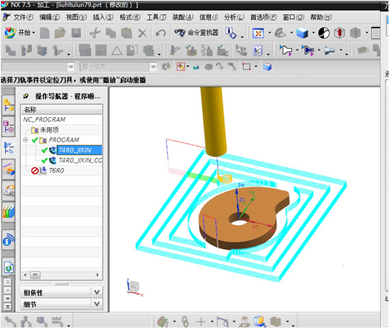Figure 2. Cam model

$E\left({x}_{1},{x}_{2}\right)=\frac{1000{x}_{1}{x}_{2}×Z}{\pi ×d×L}$ (7)

$T\left({x}_{1},{x}_{2}\right)={C}_{{}_{Z}}/{a}_{p}^{\gamma }{x}_{1}^{\alpha }{x}_{2}^{\beta }$ (8)

$C\left({x}_{1},{x}_{2}\right)={t}_{m}\cdot M+{t}_{ct}\cdot \frac{{t}_{m}}{T\left({x}_{1},{x}_{2}\right)}\cdot M+\frac{{t}_{m}}{T\left({x}_{1},{x}_{2}\right)}\cdot {C}_{t}+{t}_{ot}\cdot M$ (9)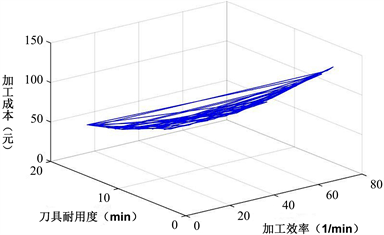Figure 3. Pareto solution set of cam cutting parametersTable 1. Optimization results of camTable 2. Selected machining parameters based on experience of cam

4. 最优解决策

4.1. 确定矛盾

4.2. 确定问题解决原理

4.3. Pareto最优解的理性决策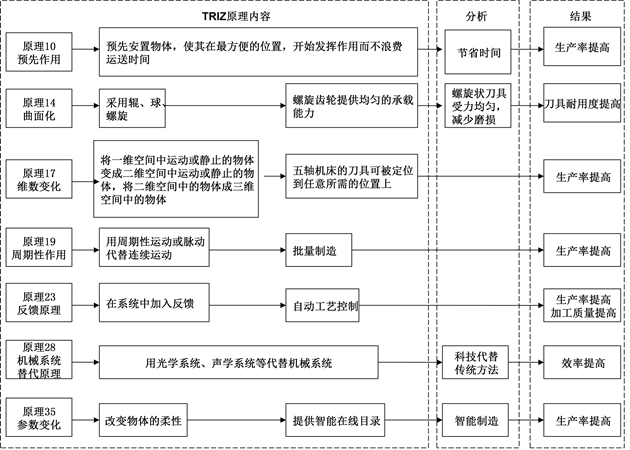Figure 4. Analysis process based on TRIZ

5. 加工实验验证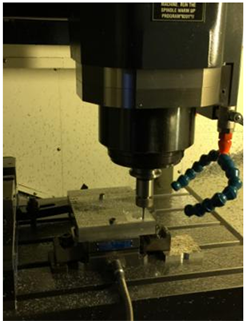Figure 5. Machining test process of camFigure 6. Machined surface roughness values of three groups parameters of camTable 4. Comparison between theoretical and actual measurement value of cam machining experiment

6. 结论

NOTES

*通讯作者。

  高亮, 杨扬, 李新宇. 数控加工参数优化的研究现状与进展[J]. 航空制造技术, 2010(22): 48-51.  张臣. 基于仿真数据的数控铣削加工多目标变参数优化[J]. 计算机辅助设计与图形学学报, 2005, 117(15): 1039-1045.  李聪波, 崔龙国, 刘飞, 等. 面向高效低碳的数控加工参数多目标优化模型[J]. 机械工程学报, 2013, 49(9): 87-96.  李倩, 詹浩, 朱军. 基于Pareto遗传算法的机翼多目标优化设计研究[J]. 西北工业大学学报, 2010, 28(1): 134-137.  段明德, 李言, 冯浩亮, 等. 高速精密数控车床床鞍结构参数多目标优化[J]. 计算机集成制造系统, 2015, 21(11): 2929-2936.  朱明放, 唐常杰, 代术成, 等. 基于基因表达式编程优化PID调节器参数[J]. 中山大学学报(自然科学版), 2008, 47(5): 18-22.  范超, 于跃, 顾宏. 基于Pareto蚁群算法的MVB周期轮询表优化设计[J]. 大连理工大学学报, 2015, 55(3): 319-325.  周志恒, 张超勇, 谢阳, 等. 数控车床切削参数的能量效率优化[J]. 计算机集成制造系统, 2015, 21(9): 2410-2418.  邓涛, 林椿松, 李亚南, 等. 基于Pareto原理的HEV能量控制参数NSGA-Ⅱ多目标优化的研究[J]. 汽车工程, 2016, 38(5): 531-537.  Ma, L.J., Gong, Y.D. and Chen, X.H. (2014) Study on Surface Roughness Model and Surface Forming Mechanism of Ceramics in Quick Point Grinding Mechanism of Ceramics in Quick Point Grinding. International Journal of Machine Tools and Manufacture, 77, 82-92.  王海艳, 秦旭达, 任成祖. 基于Pareto遗传算法的螺旋铣加工参数优化[J]. 中国机械工程, 2012, 23(17): 2058-2061.  付敏,范德林, 李锐. 基于TRIZ和AD的集成创新设计模型及其应用[J]. 机械设计, 2014(3): 10-14.  王学鹏, 成思源, 周金平, 等. TRIZ工具的简化研究及应用[J]. 机床与液压, 2014(15): 130-132.  武美萍, 翟建军, 廖文和. 数控加工切削参数优化研究[J]. 中国机械工程, 2004, 15(3): 235-237.  刘伟, 王太勇. 基于Pareto遗传算法的切削用量优化研究[J]. 农业机械学报, 2011, 42(2): 220-224, 234.  杨广勇, 王育民. 金属切削原理与刀具[M]. 北京: 北京理工大学出版社, 1994.  Asokan, P., Saravanan, R. and Vijayakuman, K. (2003) Machining Parameters Optimization for Turning Cylindrical Stock into a Continuous Finished Profile Using Genetic Algorithm (GA) and Simulated Annealing(SA). The International Journal of Advanced Manufacturing Technology, 21, 1-9. https://doi.org/10.1007/s001700300000  倪为国, 潘延华. 铣削刀具技术及应用实例[M]. 北京: 化学工业出版社, 2007.  Wang, H., Qin, X., Ren, C., et al. (2012) Prediction of Cutting Forces in Helical Milling Process. International Journal of Advanced Manufacturing Technology, 58, 849-859. https://doi.org/10.1007/s00170-011-3435-y  Mastroddi, F. and Gemma, S. (2013) Analysis of Pareto Frontiers for Multi-disciplinary Design Optimization of Aircraft. Aerospace Science and Technology, 28, 40-55. https://doi.org/10.1016/j.ast.2012.10.003  聂惠娟. 创新技法与TRIZ的应用研究[J]. 机械设计与制造, 2007(10): 222-223.  檀润华. TRIZ及应用－技术创新过程与方法[M]. 北京: 高等教育出版社, 2010.